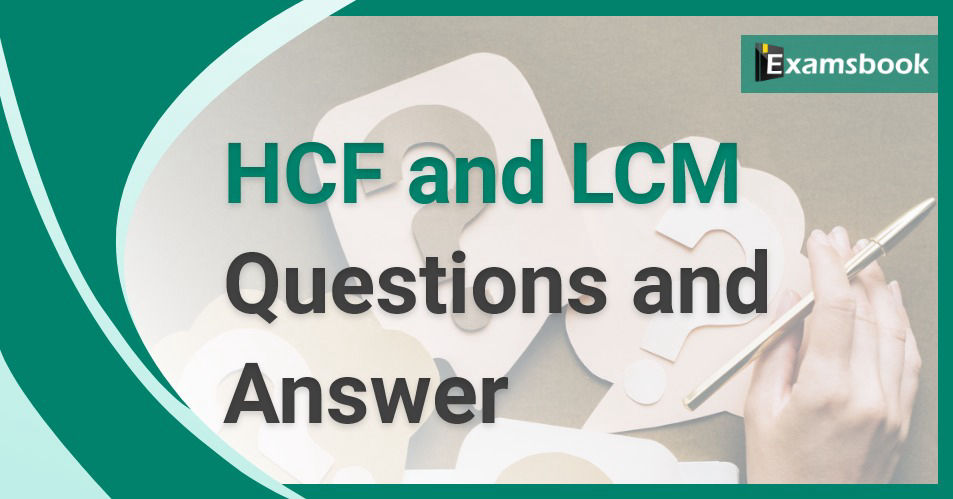• Save

# HCF and LCM Questions and Answers for Practice

Vikram Singh9 months ago 2.4K ViewsIn maths, the full form of LCM is Least Common Factor, whereas the full form of HCF is Greatest Common Factor. HCF defines the greatest factor that exists between two or more given numbers, while L.C.M. It defines the smallest number which is completely divisible by two or more numbers.

HCF and LCM is a topic that is asked in school exams apart from competitive exams. This topic is a little easier than other topics. If you practice these questions then you can score maximum marks on this topic. So, practice the questions given here as much as you can.

## Important HCF and LCM Questions

Q :

The LCM of two numbers is 44 times of their HCF. The sum of the LCM and HCF is 1125. If one number is 25, then the other number is

(A) 900

(B) 800

(C) 1100

(D) 975

Q :

The H.C.F. of two numbers, each having three digits, is 17 and their L.C.M. is 714. The sum of the numbers will be:

(A) 221

(B) 731

(C) 289

(D) 391

Q :

The L.C.M. of two numbers is 20 times their H.C.F. The sum of H.C.F. and L.C.M. is 2520. If one of the number is 480, the other number is:

(A) 520

(B) 600

(C) 400

(D) 480

Q :

The sum of two numbers is 45. Their difference is 1/9 of their sum. Their L.C.M. is

(A) 100

(B) 150

(C) 200

(D) 250

Q :

If the HCF and LCM of two consecutive (positive) even numbers be 2 and 84 respectively, then the sum of the numbers is

(A) 14

(B) 34

(C) 30

(D) 26

Q :

Sum of two numbers is 384. H.C.F. of the numbers is 48. The difference of the numbers is

(A) 288

(B) 336

(C) 100

(D) 192

Q :

The product of the LCM and the HCF of two numbers is 24. If the difference of the numbers is 2, then the greater of the number is

(A) 6

(B) 8

(C) 3

(D) 4

Q :

The ratio of two numbers is 4 : 5 and their H.C.F. is 8. Then their L.C.M. is

(A) 150

(B) 160

(C) 130

(D) 140

Q :

The sum of two numbers is 216 and their HCF is 27. How many pairs of such numbers are there?

(A) 3

(B) 0

(C) 1

(D) 2

Q :

The ratio of two numbers is 3 : 4 and their HCF is 5. Their LCM is :

(A) 15

(B) 12

(C) 10

(D) 60

### Vikram Singh

Providing knowledgable questions of Reasoning and Aptitude for the competitive exams.